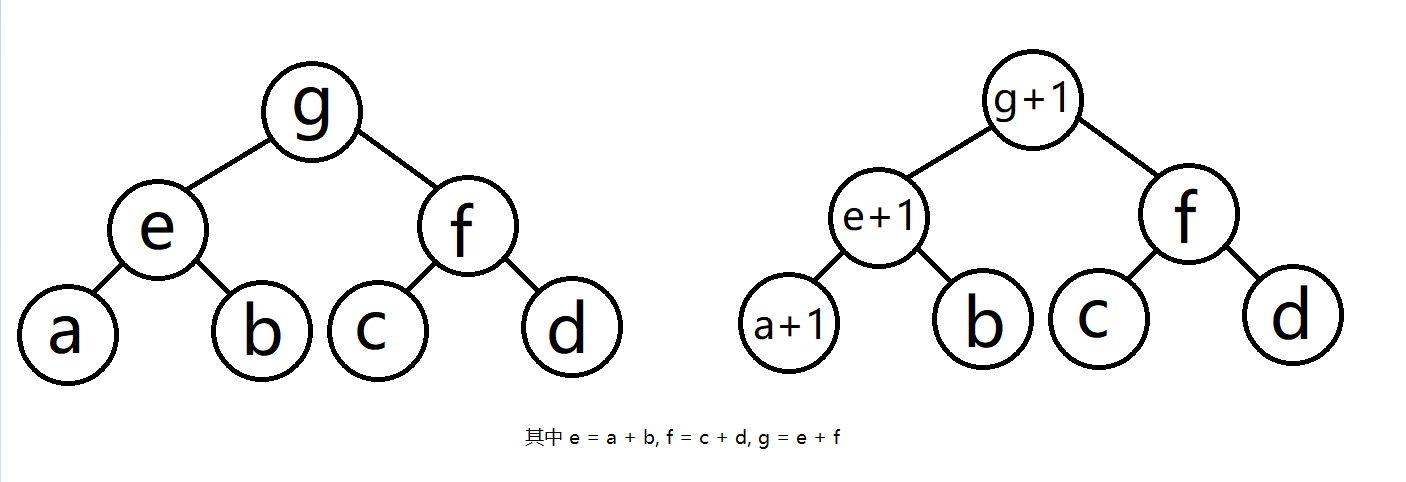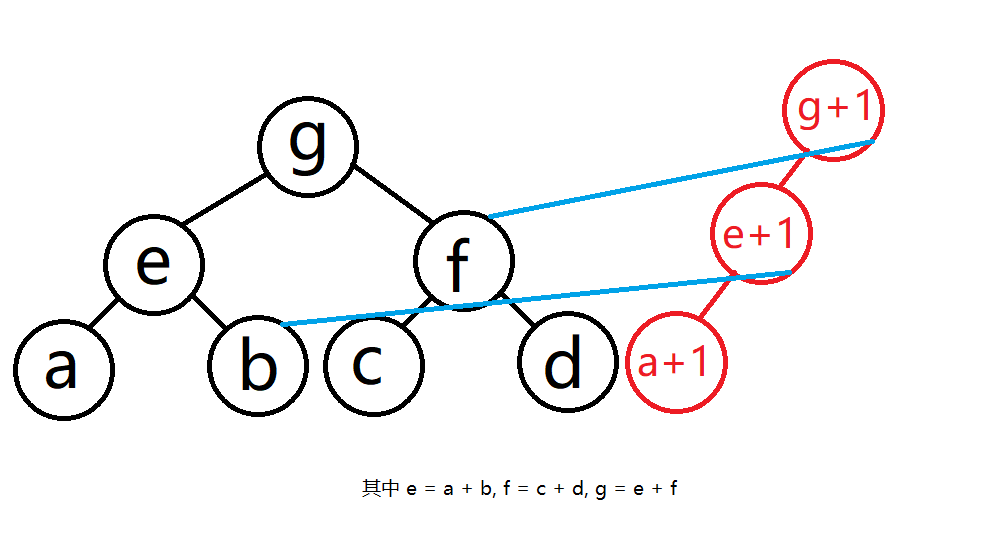线段树三(可持久化线段树&主席树)

本文代码是我临时手打的,没有测试,并不保证细节上的正确性const int maxn = 2e5;
struct node
{
int l, r, val;
}tr[maxn * 40 + 5]; // 一般都是*40 其实可以算,不过一般不会卡空间,所以多开点也无所谓
int root[maxn + 5], cnt;
int build(int l, int r) // 这里要么有返回值要么是没有返回值,但要引用调用一个变量 void build(int& pos, int l, int r) 这样写也一样
{
int now = ++ cnt; // 内存池常用用法
if(l != r)
{
int mid = (l + r) >> 1;
tr[now].l = build(l, mid); // 需要找到他的左儿子的结点
tr[now].r = build(mid + 1, r); // 需要找到他的右儿子的结点
}
if(l == r)
{
// 可以存储一些信息,不过对于权值线段树第0个版本里面所有的值为0
}
return now; // 需要把这个返回
}
int update(int pos, int l, int r, int x) // 新建立一个结点,这个结点是根据pos所建立,所以需要将他传递过来,也就是说当前新建立的结点是和pos对应的新结点, x是叶子结点要加1的
{
int now = ++ cnt; // 开辟新节点
tr[now] = tr[pos]; // 最初这个结点是完全和他所对应的结点
tr[now].val += 1; // 这个新结点的孩子必然会多1,所以copy玩之后要加1
if(l != r)
{
int mid = (l + r) >> 1;
if(x <= mid) tr[now].l = update(tr[pos].l, l, mid, x);
else tr[now].r = update(tr[pos].r, mid + 1, r, x);
// 如果新的结点在叶子结点就不需要在新建立结点了,否则若是在左子树就改变新建立的结点的左子树,否则就改变新结点的右子树
}
return now; // 依然要返回
}
int query(int pos, int cur, int l, int r, int k) // 这个是查找区间第k大的操作,之前说过根据前缀和的性质我要将两颗线段树做差,不过实际上我只需要将一条链做差就行
{
if(l == r) return l; // 如果找到叶子节点就一定是叶子节点对应的下标
int num = tr[tr[cur].l].val - tr[tr[pos].l].val; // 我看只要看左子树的大小就可以知道我往左子树递归还是右子树递归, //这里的k是保证存在的,不然需要调用函数前加个if
int mid = (l + r) >> 1;
if(num >= k) return query(tr[pos].l, tr[cur].l, l, mid, k); // 要是num >= k那肯定会在左子树中
return query(tr[pos].r, tr[cur].r, mid + 1, r, k - num); //否则的话肯定会在右子树中,并且这里要注意在右子树中代表这已经删除了num个比他排名低的,因此在右子树中找到排名就为k-num
}
<-这是注释开始->

<-这是注释结束->